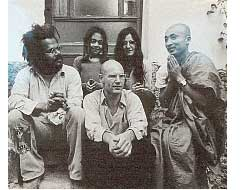This is how my attention was drawn to what I have since termed
anabelian algebraic geometry, whose starting point was exactly a study
(limited for the moment to characteristic zero) of the action of absolute
Galois groups (particularly the groups $Gal(\overline{K}/K)$, where K is an extension of finite type of the prime field) on (profinite) geometric fundamental
groups of algebraic varieties (defined over K), and more particularly (breaking with a well-established tradition) fundamental groups which are very far
from abelian groups (and which for this reason I call anabelian). Among
these groups, and very close to the group $\hat{\pi}_{0,3}$ , there is the profinite compactification of the modular group $SL_2(\mathbb{Z})$, whose quotient by its centre
$\{ \pm 1 \}$ contains the former as congruence subgroup mod 2, and can also be
interpreted as an oriented cartographic group, namely the one classifying triangulated oriented maps (i.e. those whose faces are all triangles or
monogons).The above text is taken from Alexander Grothendieck‘s visionary text Sketch of a Programme. He was interested in the permutation representations of the modular group $\Gamma = PSL_2(\mathbb{Z})$ as they correspond via Belyi-maps and his own notion of dessins d’enfants to smooth projective curves defined over $\overline{\mathbb{Q}}$. One can now study the action of the absolute Galois group $Gal(\overline{\mathbb{Q}}/\mathbb{Q})$ on these curves and their associated dessins. Because every permutation representation of $\Gamma$ factors over a finite quotient this gives an action of the absolute Galois group as automorphisms on the profinite compactification

$\hat{\Gamma} = \underset{\leftarrow}{lim}~\Gamma/N$

where the limit is taken over all finite index normal subgroups $N \triangleleft PSL_2(\mathbb{Z})$. In this way one realizes the absolute Galois group as a subgroup of the outer automorphism group of the profinite group $\hat{\Gamma}$. As a profinite group is a compact topological group one should study its continuous finite dimensional representations which are precisely those factoring through a finite quotient. In the case of $\hat{\Gamma}$ the simple continuous representations $\mathbf{simp}_c~\hat{\Gamma}$ are precisely the components of the permutation representations of the modular group. So in a sense, anabelian geometry is the study of these continuous simples together wirth the action of the absolute Galois group on it.

In noncommutative geometry we are interested in a related representation theoretic problem. We would love to know the simple finite dimensional representations $\mathbf{simp}~\Gamma$ of the modular group as this would give us all simples of the three string braid group $B_3$. So a natural question presents itself : how are these two ‘geometrical’ objects $\mathbf{simp}_c~\hat{\Gamma}$ (anabelian) and $\mathbf{simp}~\Gamma$ (noncommutative) related and can we use one to get information about the other?

This is all rather vague so far, so let us work out a trivial case to get some intuition. Consider the profinite completion of the infinite Abelian group

$\hat{\mathbb{Z}} = \underset{\leftarrow}{lim}~\mathbb{Z}/n\mathbb{Z} = \prod_p \hat{\mathbb{Z}}_p$

As all simple representations of an Abelian group are one-dimensional and because all continuous ones factor through a finite quotient $\mathbb{Z}/n\mathbb{Z}$ we see that in this case

$\mathbf{simp}_c~\hat{\mathbb{Z}} = \mu_{\infty}$

is the set of all roots of unity. On the other hand, the simple representations of $\mathbb{Z}$ are also one-dimensional and are determined by the image of the generator so

$\mathbf{simp}~\mathbb{Z} = \mathbb{C} – { 0 } = \mathbb{C}^*$

Clearly we have an embedding $\mu_{\infty} \subset \mathbb{C}^*$ and the roots of unity are even dense in the Zariski topology. This might look a bit strange at first because clearly all roots of unity lie on the unit circle which ‘should be’ their closure in the complex plane, but that’s because we have a real-analytic intuition. Remember that the Zariski topology of $\mathbb{C}^*$ is just the cofinite topology, so any closed set containing the infinitely many roots of unity should be the whole space!

Let me give a pedantic alternative proof of this (but one which makes it almost trivial that a similar result should be true for most profinite completions…). If $c$ is the generator of $\mathbb{Z}$ then the different conjugacy classes are precisely the singletons $c^n$. Now suppose that there is a polynomial $a_0+a_1x+\ldots+a_mx^m$ vanishing on all the continuous simples of $\hat{\mathbb{Z}}$ then this means that the dimensions of the character-spaces of all finite quotients $\mathbb{Z}/n\mathbb{Z}$ should be bounded by $m$ (for consider $x$ as the character of $c$), which is clearly absurd.

Hence, whenever we have a finitely generated group $G$ for which there is no bound on the number of irreducibles for finite quotients, then morally the continuous simple space for the profinite completion

$\mathbf{simp}_c~\hat{G} \subset \mathbf{simp}~G$

should be dense in the Zariski topology on the noncommutative space of simple finite dimensional representations of $G$. In particular, this should be the case for the modular group $PSL_2(\mathbb{Z})$.

There is just one tiny problem : unlike the case of $\mathbb{Z}$ for which this space is an ordinary (ie. commutative) affine variety $\mathbb{C}^*$, what do we mean by the “Zariski topology” on the noncommutative space $\mathbf{simp}~PSL_2(\mathbb{Z})$ ? Next time we will clarify what this might be and show that indeed in this case the subset

$\mathbf{simp}_c~\hat{\Gamma} \subset \mathbf{simp}~\Gamma$

will be a Zariski closed subset!